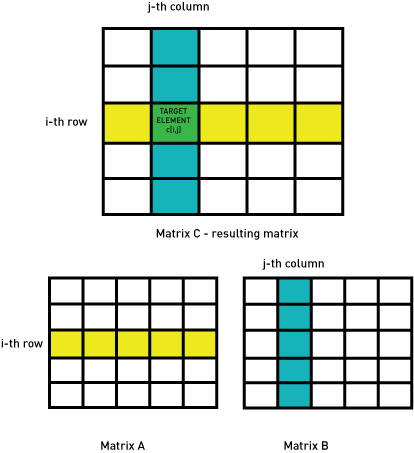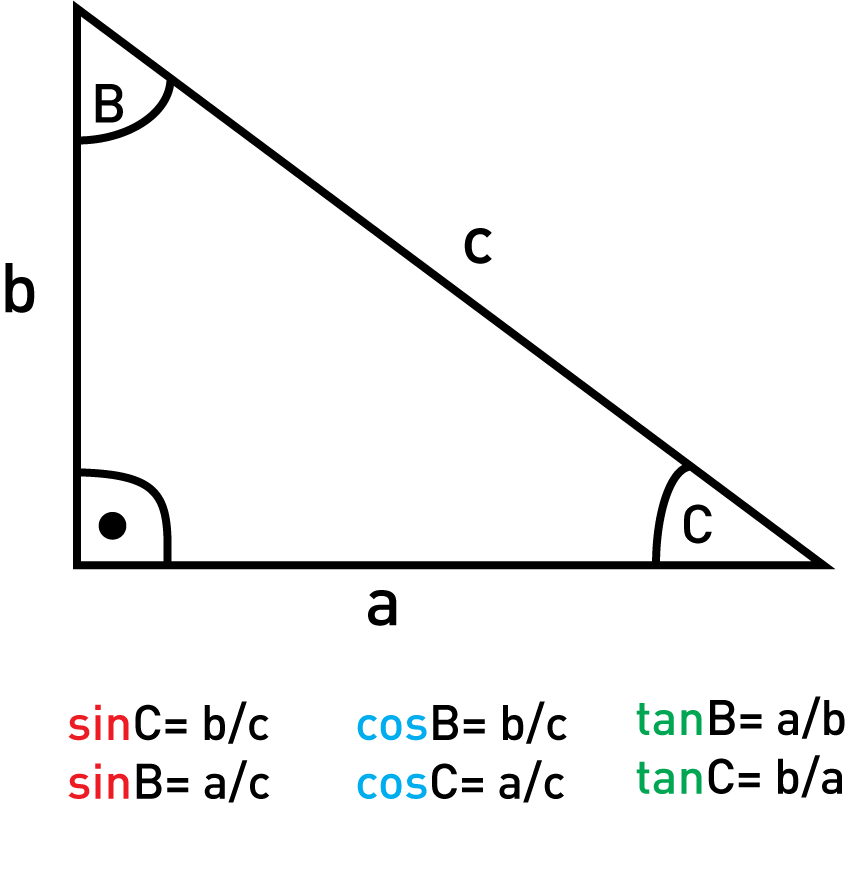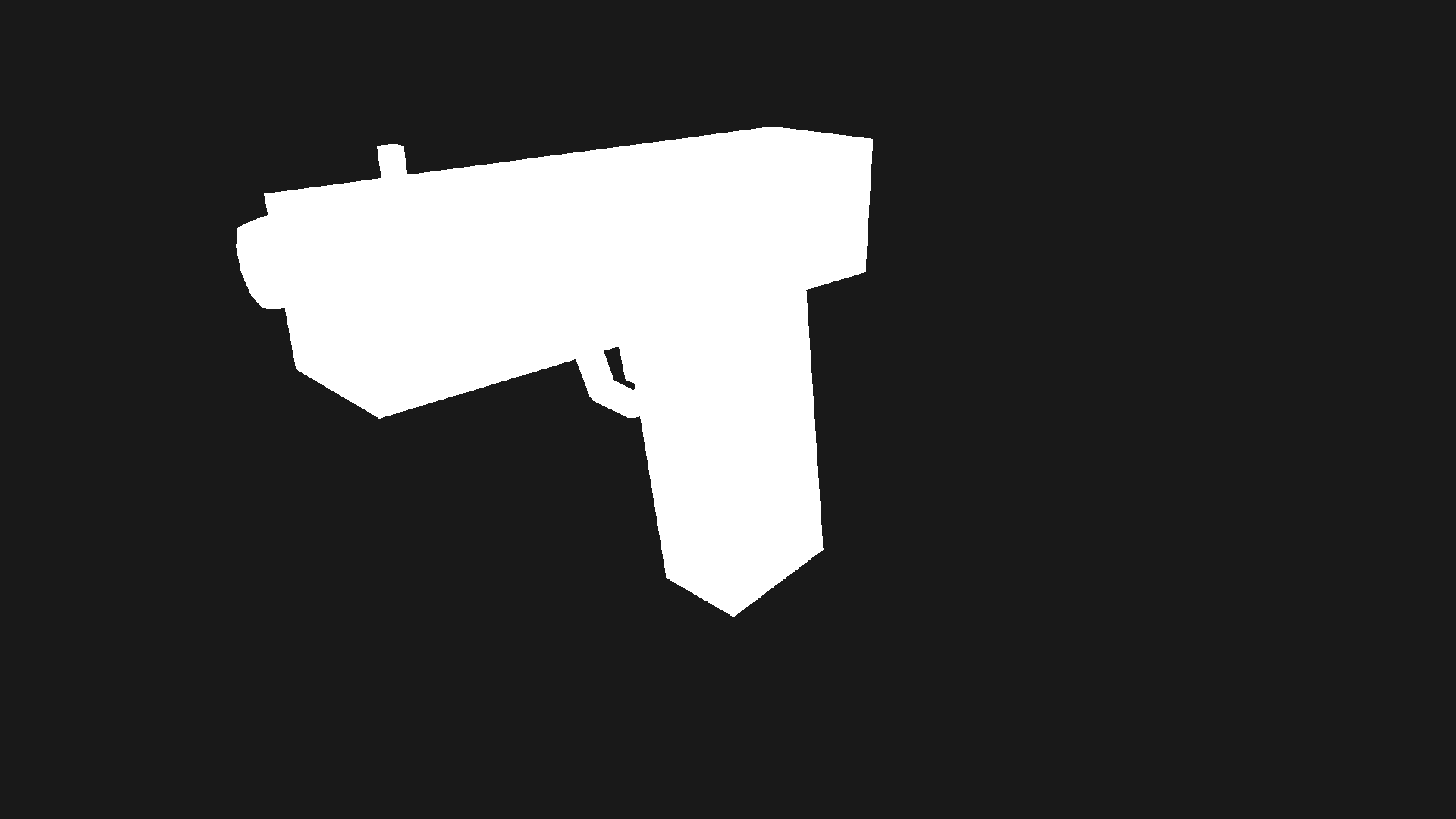Understanding OpenGL through Python - Stack Abuse

# Understanding OpenGL through Python### Introduction

Following this article by Muhammad Junaid Khalid, where basic OpenGL concepts and setup was explained, now we'll be looking at how to make more complex objects and how to animate them.

OpenGL is very old, and you won't find many tutorials online on how to properly use it and understand it because all the top dogs are already knee-deep in new technologies.

To understand modern OpenGL code, you have to first understand the ancient concepts that were written on stone tablets by the wise Mayan game developers.

In this article, we'll jump into several fundamental topics you'll need to know:

In the last section we'll take a look at how to actually use OpenGL with the Python libraries PyGame and PyOpenGL.

In the next article we'll take a deeper look at how to use OpenGL with Python and the libraries mentioned above.

### Basic Matrix Operations

To properly be able to use many of the functions in OpenGL, we'll need some geometry.

Every single point in space can be represented with Cartesian coordinates. Coordinates represent any given point's location by defining it's X, Y and Z values.

We'll be practically using them as 1x3 matrices, or rather 3-dimensional vectors (more on matrices later on).

Here are examples of some coordinates:

a and b being points in space, their x-coordinates being 5 and 9 respectively, y-coordinates being 3 and 1, and so on.

In computer graphics, more often than not, homogeneous coordinates are utilized instead of regular old Cartesian coordinates. They're basically the same thing, only with an additional utility parameter, which for the sake of simplicity we'll say is always 1.

So if the regular coordinates of a are (5,3,4), the corresponding homogeneous coordinates would be (5,3,4,1). There's a lot of geometric theory behind this, but it isn't really necessary for this article.

Next, an essential tool for representing geometric transformations are matrices. A matrix is basically a two-dimensional array (in this case of size n*n, it's very important for them to have the same number of rows and columns).

Now matrix operations are, more often than not, pretty straightforward, like addition, subtraction, etc. But of course the most important operation has to be the most complicated one - multiplication. Let's take a look at basic matrix operation examples:

Now, as all math tends to do, it gets relatively complicated when you actually want something practical out of it.

The formula for matrix multiplication goes as follows:

$$c[i,j] = \sum_{k=1}^{n}a[i,k]*b[k,j]$$

c being the resulting matrix, a and b being the multiplicand and the multiplier.

There's a simple explanation for this formula, actually. Every element can be constructed by summing the products of all the elements in the i-th row and the j-th column. This is the reason why in a[i,k], the i is fixed and the k is used to iterate through the elements of the corresponding row. Same principle can be applied to b[k,j].

Knowing this, there's an additional condition that needs to be fulfilled for us to be able to use matrix multiplication. If we want to multiply matrices A and B of dimensions a*b and c*d. The number of elements in a single row in the first matrix (b) has to be the same as the number of elements in a column in the second matrix (c), so that the formula above can be used properly.

A very good way of visualizing this concept is highlighting the rows and columns who's elements are going to be utilized in the multiplication for a given element. Imagine the two highlighted lines over each other, as if they're in the same matrix.

The element where they intercept is the position of the resulting element of the summation of their products:Matrix multiplication is so important because if we want to explain the following expression in simple terms: A*B (A and B being matrices), we would say:

We are transforming A using B.

This is why matrix multiplication is the quintessential tool for transforming any object in OpenGL or geometry in general.

The last thing you need to know about matrix multiplication is that it has a neutral. This means there is a unique element (matrix in this case) E which when multiplied with any other element A doesn't change A's value, that is:

$$(!\exists{E}\ \ \forall{A})\ E*A=A$$

The exclamation point in conjunction with the exists symbol means: A unique element E exists which...

In case of multiplication with normal integers, E has the value of 1. In case of matrices, E has the following values in normal Cartesian (E1) and homogeneous coordinates (E2) respectively:

${E}_{1}=\left[\begin{array}{ccc}1& 0& 0\\ 0& 1& 0\\ 0& 0& 1\end{array}\right]{E}_{2}=\left[\begin{array}{cccc}1& 0& 0& 0\\ 0& 1& 0& 0\\ 0& 0& 1& 0\\ 0& 0& 0& 1\end{array}\right]$

Every single geometric transformation has it's own unique transformation matrix that has a pattern of some sort, of which the most important are:

• Translation
• Scaling
• Reflection
• Rotation
• Sheering

#### Translation

Translation is the act of literally moving an object by a set vector. The object that's affected by the transformation doesn't change its shape in any way, nor does it change its orientation - it's just moved in space (that's why translation is classified as a movement transformation).

Translation can be described with the following matrix form:

$T=\left[\begin{array}{cccc}1& 0& 0& {t}_{x}\\ 0& 1& 0& {t}_{y}\\ 0& 0& 1& {t}_{z}\\ 0& 0& 0& 1\end{array}\right]$

The t-s represents by how much the object's x,y and z location values will be changed.

So, after we transform any coordinates with the translation matrix T, we get:

$$[x,y,z]*T=[t_x+x,t_y+y,t_z+z]$$

Translation is implemented with the following OpenGL function:

void glTranslatef(GLfloat tx, GLfloat ty, GLfloat tz);


As you can see, if we know the form of the translation matrix, understanding the OpenGL function is very straightforward, this is the case with all OpenGL transformations.

Don't mind the GLfloat, it's just a clever data type for OpenGL to work on multiple platforms, you can look at it like this:

typedef float GLfloat;
typedef double GLdouble;
typedef someType GLsomeType;


This is a necessary measure because not all systems have the same storage space for a char, for example.

#### Rotation

Rotation is bit more complicated transformation, because of the simple fact it's dependent on 2 factors:

• Pivot: Around what line in 3D space (or point in 2D space) we'll be rotating
• Amount: By how much (in degrees or radians) we'll be rotating

Because of this, we first need to define rotation in a 2D space, and for that we need a bit of trigonometry.

Here's a quick reference:

## Better understand your data with visualizations.

•  30-day no-questions money-back guarantee
•  Beginner to Advanced
•  Updated regularly (latest update June 2021)
•  Updated with bonus resources and guidesThese trigonometric functions can only be used inside a right-angled triangle (one of the angles has to be 90 degrees).

The base rotation matrix for rotating an object in 2D space around the vertex (0,0) by the angle A goes as follows:

$\left[\begin{array}{ccc}cosA& -sinA& 0\\ sinA& cosA& 0\\ 0& 0& 1\end{array}\right]$

Again, the 3rd row and 3rd column are just in case we want to stack translation transformations on top of other transformations (which we will in OpenGL), it's ok if you don't fully grasp why they're there right now. Things should clear up in the composite transformation example.

This was all in 2D space, now let's move on to 3D space. In 3D space we need to define a matrix that can rotate an object around any line.

As a wise man once said: "Keep it simple and stupid!" Fortunately, math magicians did for once keep it simple and stupid.

Every single rotation around a line can be broken down into a few transformations:

• Rotation around the x axis
• Rotation around the y axis
• Rotation around the z axis
• Utility translations (which will be touched upon later)

So, the only three things we need to construct for any 3D rotation are matrices that represent rotation around the x, y, and z axis by an angle A:

${R}_{x}=\left[\begin{array}{cccc}1& 0& 0& 0\\ 0& cosA& -sinA& 0\\ 0& sinA& cosA& 0\\ 0& 0& 0& 1\end{array}\right]{R}_{y}=\left[\begin{array}{cccc}cosA& 0& sinA& 0\\ 0& 1& 0& 0\\ -sinA& 0& cosA& 0\\ 0& 0& 0& 1\end{array}\right]{R}_{z}=\left[\begin{array}{cccc}cosA& -sinA& 0& 0\\ sinA& cosA& 0& 0\\ 0& 0& 1& 0\\ 0& 0& 0& 1\end{array}\right]$

3D rotation is implemented with the following OpenGL function:

void glRotatef(GLfloat angle, GLfloat x, GLfloat y, GLfloat z);

• angle: angle of rotation in degrees (0-360)
• x,y,z: vector around which the rotation is executed

#### Scaling

Scaling is the act of multiplying any dimension of the target object by a scalar. This scalar can be <1 if we want to shrink the object, and it can be >1 if we want to enlarge the object.

Scaling can be described with the following matrix form:

$S=\left[\begin{array}{cccc}{s}_{x}& 0& 0& 0\\ 0& {s}_{y}& 0& 0\\ 0& 0& {s}_{z}& 0\\ 0& 0& 0& 1\end{array}\right]$

sx, sy, sz are the scalars that are multiplied with the x, y, and z values of the target object.

After we transform any coordinates with the scaling matrix S we get:

$\left[x,y,z\right]\ast S=\left[{s}_{x}\ast x,{s}_{y}\ast y,{s}_{z}\ast z\right]$

This transformation is particularly useful when scaling an object by factor k (this means the resulting object is two times bigger), this is achieved by setting sx=sy=sz=k:

$\left[x,y,z\right]\ast S=\left[{s}_{x}\ast x,{s}_{y}\ast y,{s}_{z}\ast z\right]$

A special case of scaling is known as reflection. It's achieved by setting either sx, sy, or sz to -1. This just means we invert the sign of one of the object's coordinates.

In simpler terms, we put the object on the other side of the x, y, or z axis.

This transformation can be modified to work for any plain of reflection, but we don't really need it for now.

void glScalef(GLfloat sx, GLfloat sy, GLfloat sz);


### Composite Transformations

Composite transformations are transformations which consist of more than 1 basic transformation (listed above). Transformations A and B are combined by matrix multiplying the corresponding transformation matrices M_a and M_b.

This may seem like very straightforward logic, however there are some things that can be confusing. For example:

• Matrix multiplication is not commutable:
• Every single one of these transformations has an inverse transformation. An inverse transformation is a transformation that cancels out the original one:
• When we want to make an inverse of a composite transformation, we have to change the order of elements utilized:
$\left(A\ast B\ast C{\right)}^{-1}={C}^{-1}\ast {B}^{-1}\ast {A}^{-1}$

The point is - the topological order of matrix utilization is very important, just like ascending to a certain floor of a building.

If you're on the first floor, and you want to get to the fourth floor, first you need to go to the third floor and then to the fourth.

But if you want to descend back to the second floor, you would then have to go to the third floor and then to the second floor (in reverse topological order).

### Transformations that Involve a Referral Point

As previously mentioned, when a transformation has to be done relative to a specific point in space, for example rotating around a referral point A=(a,b,c) in 3D space, not the origin O=(0,0,0), we need to turn that referral point A into O by translating everything by T(-a,-b,-c).

Then we can do any transformation we need to do, and when we're done, translate everything back by T(a,b,c), so that the original origin O again has the coordinates (0,0,0).

The matrix form of this example is:

$T\ast M\ast {T}^{-1}=\left[\begin{array}{cccc}1& 0& 0& -a\\ 0& 1& 0& -b\\ 0& 0& 1& -c\\ 0& 0& 0& 1\end{array}\right]\ast M\ast \left[\begin{array}{cccc}1& 0& 0& a\\ 0& 1& 0& b\\ 0& 0& 1& c\\ 0& 0& 0& 1\end{array}\right]$

Where M is the transformation we wish to do on an object.

The whole point to learning these matrix operations is so that you can fully understand how OpenGL works.

### Modeling Demonstration

With all of that out of the way, let's take a look at a simple modeling demonstration.

In order to do anything with OpenGL through Python, we'll use two modules - PyGame and PyOpenGL:

$python3 -m pip install -U pygame --user$ python3 -m pip install PyOpenGL PyOpenGL_accelerate


Because it's redundant to unload 3 books worth of graphics theory on yourself, we'll be using the PyGame library. It will essentially just shorten the process from project initialization to actual modeling and animating.

To start off, we need to import everything necessary from both OpenGL and PyGame:

import pygame as pg
from pygame.locals import *

from OpenGL.GL import *
from OpenGL.GLU import *


In the following example, we can see that to model unconventional object, all we need to know is how the complex object can be broken down into smaller and simpler pieces.

Because we still don't know what some of these functions do, I'll give some surface level definitions in the code itself, just so you can see how OpenGL can be used. In the next article, all of these will be covered in detail - this is just to give you a basic idea of how working with OpenGL looks like:

def draw_gun():
# Setting up materials, ambient, diffuse, specular and shininess properties are all
# different properties of how a material will react in low/high/direct light for
# example.
ambient_coeffsGray = [0.3, 0.3, 0.3, 1]
diffuse_coeffsGray = [0.5, 0.5, 0.5, 1]
specular_coeffsGray = [0, 0, 0, 1]
glMaterialfv(GL_FRONT, GL_AMBIENT, ambient_coeffsGray)
glMaterialfv(GL_FRONT, GL_DIFFUSE, diffuse_coeffsGray)
glMaterialfv(GL_FRONT, GL_SPECULAR, specular_coeffsGray)
glMateriali(GL_FRONT, GL_SHININESS, 1)

# OpenGL is very finicky when it comes to transformations, for all of them are global,
# so it's good to seperate the transformations which are used to generate the object
# from the actual global transformations like animation, movement and such.
# The glPushMatrix() ----code----- glPopMatrix() just means that the code in between
# these two functions calls is isolated from the rest of your project.
# Even inside this push-pop (pp for short) block, we can use nested pp blocks,
# which are used to further isolate code in it's entirety.
glPushMatrix()

glPushMatrix()
glTranslatef(3.1, 0, 1.75)
glRotatef(90, 0, 1, 0)
glScalef(1, 1, 5)
glScalef(0.2, 0.2, 0.2)
glutSolidTorus(0.2, 1, 10, 10)
glPopMatrix()

glPushMatrix()
glTranslatef(2.5, 0, 1.75)
glScalef(0.1, 0.1, 1)
glutSolidCube(1)
glPopMatrix()

glPushMatrix()
glTranslatef(1, 0, 1)
glRotatef(10, 0, 1, 0)
glScalef(0.1, 0.1, 1)
glutSolidCube(1)

glPopMatrix()

glPushMatrix()
glTranslatef(0.8, 0, 0.8)
glRotatef(90, 1, 0, 0)
glScalef(0.5, 0.5, 0.5)
glutSolidTorus(0.2, 1, 10, 10)
glPopMatrix()

glPushMatrix()
glTranslatef(1, 0, 1.5)
glRotatef(90, 0, 1, 0)
glScalef(1, 1, 4)
glutSolidCube(1)
glPopMatrix()

glPushMatrix()
glRotatef(8, 0, 1, 0)
glScalef(1.1, 0.8, 3)
glutSolidCube(1)
glPopMatrix()

glPopMatrix()

def main():
# Initialization of PyGame modules
pg.init()
# Initialization of Glut library
glutInit(sys.argv)
# Setting up the viewport, camera, backgroud and display mode
display = (800,600)
pg.display.set_mode(display, DOUBLEBUF|OPENGL)
glClearColor(0.1,0.1,0.1,0.3)
gluPerspective(45, (display/display), 0.1, 50.0)
gluLookAt(5,5,3,0,0,0,0,0,1)

glTranslatef(0.0,0.0, -5)
while True:
# Listener for exit command
for event in pg.event.get():
if event.type == pg.QUIT:
pg.quit()
quit()

# Clears the screen for the next frame to be drawn over
glClear(GL_COLOR_BUFFER_BIT|GL_DEPTH_BUFFER_BIT)
############## INSERT CODE FOR GENERATING OBJECTS ##################
draw_gun()
####################################################################
# Function used to advance to the next frame essentially
pg.display.flip()
pg.time.wait(10)


This whole bunch of code yields us:### Conclusion

OpenGL is very old, and you won't find many tutorials online on how to properly use it and understand it because all the top dogs are already knee-deep in new technologies.

To properly use OpenGL, one needs to grasp the basic concepts in order to understand the implementations through OpenGL functions.

In this article, we've covered basic matrix operations (translation, rotation, and scaling) as well as composite transformations and transformations that involve a referral point.

In the next article, we'll be using PyGame and PyOpenGL to initialize a project, draw objects, animate them, etc.!

Last Updated: February 2nd, 2020

## Improve your dev skills!

Get tutorials, guides, and dev jobs in your inbox.

No spam ever. Unsubscribe at any time. Read our Privacy Policy.

# Prepping for an interview?

• Improve your skills by solving one coding problem every day
• Get the solutions the next morning via email
• Practice on actual problems asked by top companies, like:

© 2013-2021 Stack Abuse. All rights reserved.